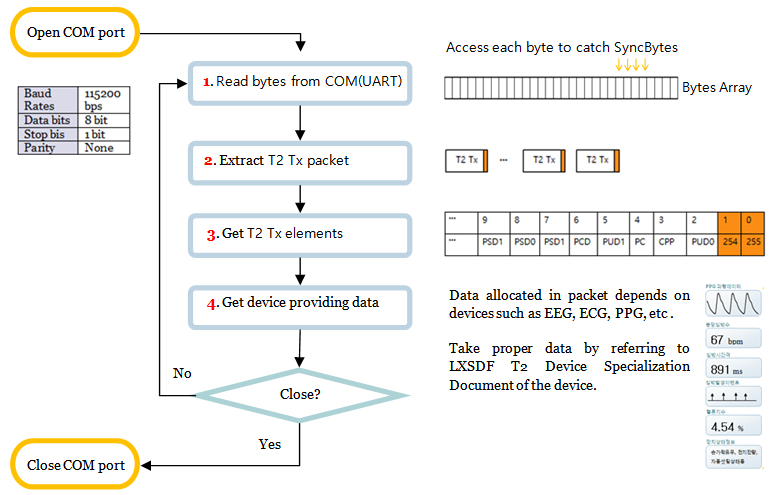The following picture shows the programming flow of host side.

First of all, open com port in host. After that,

1. Read bytes from COM : Read bytes received from com port(UART) in order.
2. Extract T2 Tx packet : Catch “SyncBytes” (it is placed in order of 255,254) which means packet’s start spot from byte row separates data up and then abstract data elements in the packet from
3. Get T2 Tx elements : Get all the T2 Tx packet elements.
4. Get device providing data : Data allocation situation depends on each device. Refer to LXSDF T2 Device Specialization Documents of the device.

The following picture shows the whole program structure of host side.### Code Example. T2 Tx packet extracting & T2 Tx elements taking.

This code shows the example of  processing code of phase 2 and 3 in the picture. (C#code). This example just use simple general function so coding method is the same regardless of the language.

```        // transmit the data received by serial with the function by 1 byte  in order .
// processing  in the function : Find the sync spot and abstract data by each packet TX Index .

bool Sync_After = false;
byte Packet_TX_Index = 0;
byte Data_Prev = 0; // PREVALUE

byte PUD0 = 0;
byte CRD_PUD2_PCDT = 0;
byte PUD1 = 0;
byte PacketCount = 0;
byte PacketCyclicData = 0;
byte psd_idx = 0;

int Parsing_LXSDFT2(byte data_crnt)
{
int retv = 0;

if (Data_Prev == 255 && data_crnt == 254)// Found sync spot.
{
Sync_After = true;
Packet_TX_Index = 0;                  // Initialize  packet  TX Index  0.
}

Data_Prev = data_crnt;                   // receive the present value as pre value.

if (Sync_After == true)                  // only task after discovering sync.
{
Packet_TX_Index++;                    // increase TX Index 1. The spot where is discovered 254 is 1. Whenever receiving 1 byte as serial, it increases 1.
if (Packet_TX_Index > 1)              // only task over TX Index  2.
{
if (Packet_TX_Index == 2)            // occupied TX Index2 PUD0.
PUD0 = data_crnt;
else if (Packet_TX_Index == 3)       // occupied TX Index 3 CRD, PUD2, PCD Type
CRD_PUD2_PCDT = data_crnt;
else if (Packet_TX_Index == 4)       // occupied TX Index 4  PC.
PacketCount = data_crnt;
else if (Packet_TX_Index == 5)       // occupied TX Index 5  PUD1.
PUD1 = data_crnt;
else if (Packet_TX_Index == 6)       // occupied TX Index 6 PCD(Packet cyclic data) .
PacketCyclicData = data_crnt;
else if (Packet_TX_Index > 6)        // Stream data(wave-pattern data) enters one each in order in more than TX Index 7. the data procedure to receive ->  it is recorded in order of Ch 1 high byte , Ch 2 high byte low byte..
{
psd_idx = (byte)(Packet_TX_Index - 7); // Packet Stream Data arrangement Index.
PacketStreamData[psd_idx] = data_crnt; // crnt data is occupied in order and stream data is only occupied.
if (Packet_TX_Index == (Ch_Num * 2 * Sample_Num + 6)) // Channel number  x 2( 2bytes occupation) x Sample capacity + 6(Index value before the front section of wave-pattern data) is the end of one packet.
{
Sync_After = false; // Be false to search sync spot again.
retv = 1; // If Passing of 1 packet unit is finished, it will be returned.
}
}
} //if (Packet_TX_Index > 1)

}
return retv; //If 1  packet is finished, others return 0.
}
```